Amazon Web Services 文档中描述的 Amazon Web Services 服务或功能可能因区域而异。要查看适用于中国区域的差异，请参阅中国的 Amazon Web Services 服务入门

# 了解坐标系和传感器融合

• 当您 时是必需的在世界坐标系或全局参照系中提供输入数据。

• 什么是世界坐标，以及如何将点云数据转换为世界坐标系？

• 在使用传感器融合时，如何使用传感器和摄像机外部矩阵提供姿势数据？

## 在世界坐标系中使用点云数据

### 什么是世界坐标系？

WCS 或全局参照系是固定的通用坐标系，车辆和传感器坐标系放置在该坐标系中。例如，如果从两个传感器中收集多个点云帧而导致它们位于不同的坐标系中，可以使用 WCS 将这些点云帧中的所有坐标转换为单个坐标系，其中，所有帧具有相同的原点 (0,0,0)。这种变换是通过以下方法完成的：使用平移向量将每个帧的原点平移到 WCS 的原点，并使用旋转矩阵将三个轴（通常为 x、y 和 z）旋转到正确的方向。这种刚体变换称为齐次变换

### 将 3D 点云数据转换为 WCS

Ground Truth 假设点云数据已变换为所选的参照坐标系。例如，您可以选择传感器（例如 LiDAR）的参照坐标系以作为全局参照坐标系。您也可以从不同传感器中获取点云，并将其从传感器的视图变换为车辆的参照坐标系视图。您可以使用传感器的外部矩阵（由旋转矩阵和平移向量组成）将点云数据转换为 WCS 或全局参照系。

``LiDAR_extrinsic = [R T;0 0 0 1]``

``````import pykitti
import numpy as np

basedir = '/Users/nameofuser/kitti-data'
date = '2011_09_26'
drive = '0079'

# The 'frames' argument is optional - default: None, which loads the whole dataset.
# Calibration, timestamps, and IMU data are read automatically.
# Camera and velodyne data are available via properties that create generators
# when accessed, or through getter methods that provide random access.
data = pykitti.raw(basedir, date, drive, frames=range(0, 50, 5))

# i is frame number
i = 0

# lidar extrinsic for the ith frame
lidar_extrinsic_matrix = data.oxts[i].T_w_imu

# velodyne raw point cloud in lidar scanners own coordinate system
points = data.get_velo(i)

# transform points from lidar to global frame using lidar_extrinsic_matrix
def generate_transformed_pcd_from_point_cloud(points, lidar_extrinsic_matrix):
tps = []
for point in points:
transformed_points = np.matmul(lidar_extrinsic_matrix, np.array([point, point, point, 1], dtype=np.float32).reshape(4,1)).tolist()
if len(point) > 3 and point is not None:
tps.append([transformed_points, transformed_points, transformed_points, point])

return tps

# customer transforms points from lidar to global frame using lidar_extrinsic_matrix
transformed_pcl = generate_transformed_pcd_from_point_cloud(points, lidar_extrinsic_matrix)    ``````

## 传感器融合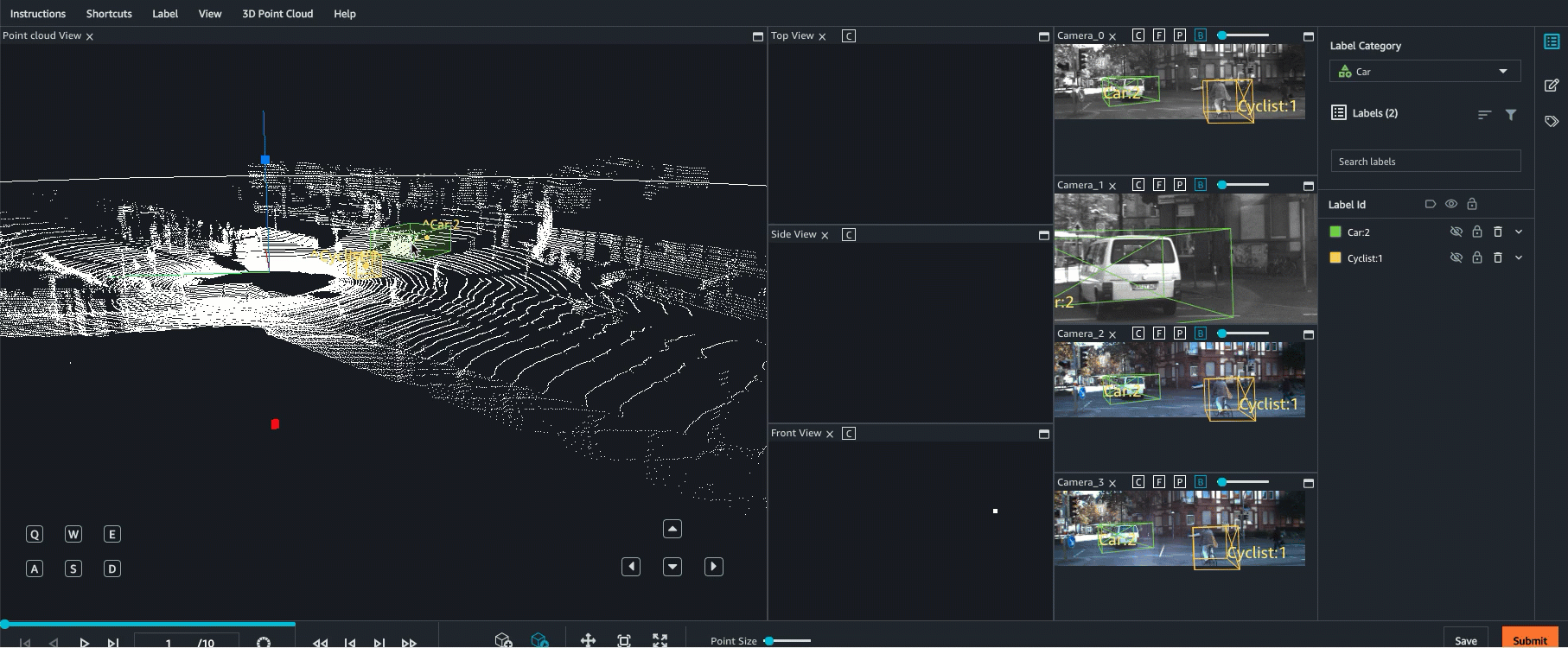### 外部矩阵

Ground Truth 使用激光雷达和摄像头计算这些外在矩阵姿势数据您提供的：`heading`（以四元数为单位：`qx``qy``qz`, 和`qw`) 和`position`(`x``y``z`)。对于车辆，通常通过世界坐标系中的车辆参照系描述方向和位置，它们称为自主车辆姿势。对于每个摄像机外部矩阵，您可以为该摄像机添加姿势信息。有关更多信息，请参阅 Pose

### Pose

``````import numpy

rotation = [[ 9.96714314e-01, -8.09890350e-02,  1.16333982e-03],
[ 8.09967396e-02,  9.96661051e-01, -1.03090934e-02],
[-3.24531964e-04,  1.03694477e-02,  9.99946183e-01]]

origin= [1.71104606e+00,
5.80000039e-01,
9.43144935e-01]

from scipy.spatial.transform import Rotation as R

# position is the origin
position = origin
r = R.from_matrix(np.asarray(rotation))

# heading in WCS using scipy
``````

1. `x`（浮点值）自主车辆、传感器或摄像机位置的 x 坐标（以米为单位）。

2. `y`（浮点值）自主车辆、传感器或摄像机位置的 y 坐标（以米为单位）。

3. `z`（浮点值）自主车辆、传感器或摄像机位置的 z 坐标（以米为单位）。

``````{
"position": {
"y": -152.77584902657554,
"x": 311.21505956090624,
"z": -10.854137529636024
}
}``````

`heading` 对象中的字段如下所示：

1. `qx`（浮点值）- 自主车辆、传感器或摄像机方向的 x 分量。

2. `qy`（浮点值）- 自主车辆、传感器或摄像机方向的 y 分量。

3. `qz`（浮点值）- 自主车辆、传感器或摄像机方向的 z 分量。

4. `qw`（浮点值）- 自主车辆、传感器或摄像机方向的 w 分量。

``````{
"qy": -0.7046155108831117,
"qx": 0.034278837280808494,
"qz": 0.7070617895701465,
"qw": -0.04904659893885366
}
}``````

## 计算方向四元数和位置

Ground Truth 必须以四元数形式提供所有方向或方位数据。四元数是与测地线球形属性一致的方向的表示形式，可用于对旋转进行近似计算。与欧拉角相比，它们的组成更简单，并且避免了万向节死锁问题。与旋转矩阵相比，它们更紧凑，并且数值稳定性和效率更高。

``````import numpy

rotation = [[ 9.96714314e-01, -8.09890350e-02,  1.16333982e-03],
[ 8.09967396e-02,  9.96661051e-01, -1.03090934e-02],
[-3.24531964e-04,  1.03694477e-02,  9.99946183e-01]]

origin = [1.71104606e+00,
5.80000039e-01,
9.43144935e-01]

from scipy.spatial.transform import Rotation as R
# position is the origin
position = origin
r = R.from_matrix(np.asarray(rotation))
# heading in WCS using scipy
``````

3D 旋转转换器之类的 UI 工具也可能是非常有用的。

``````import numpy as np

transformation
= [[ 9.96714314e-01, -8.09890350e-02,  1.16333982e-03, 1.71104606e+00],
[ 8.09967396e-02,  9.96661051e-01, -1.03090934e-02, 5.80000039e-01],
[-3.24531964e-04,  1.03694477e-02,  9.99946183e-01, 9.43144935e-01],
[              0,               0,               0,              1]]

transformation  = np.array(transformation )
rotation = transformation[0:3][0:3]
translation= transformation[0:3]

from scipy.spatial.transform import Rotation as R
# position is the origin translation
position = translation
r = R.from_matrix(np.asarray(rotation))
# heading in WCS using scipy

``````struct Quaternion
{
double w, x, y, z;
};

Quaternion ToQuaternion(double yaw, double pitch, double roll) // yaw (Z), pitch (Y), roll (X)
{
// Abbreviations for the various angular functions
double cy = cos(yaw * 0.5);
double sy = sin(yaw * 0.5);
double cp = cos(pitch * 0.5);
double sp = sin(pitch * 0.5);
double cr = cos(roll * 0.5);
double sr = sin(roll * 0.5);

Quaternion q;
q.w = cr * cp * cy + sr * sp * sy;
q.x = sr * cp * cy - cr * sp * sy;
q.y = cr * sp * cy + sr * cp * sy;
q.z = cr * cp * sy - sr * sp * cy;

return q;
}``````

## Ground Truth 传感器融合变换

### LiDAR 外部矩阵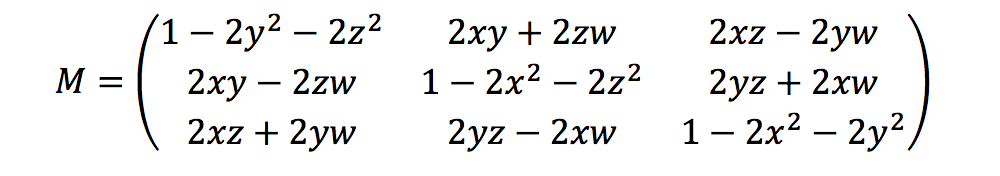``LiDAR_extrinsic = [R T;0 0 0 1]``

### 相机校准：外部矩阵、内部矩阵和畸变

#### 摄像机外部矩阵

`` camera_inverse_extrinsic = inv([Rc Tc;0 0 0 1]) #where Rc and Tc are camera pose components``

#### 内部矩阵和畸变

Ground Truth 可以纠正两种类型的失真：径向失真和切向失真。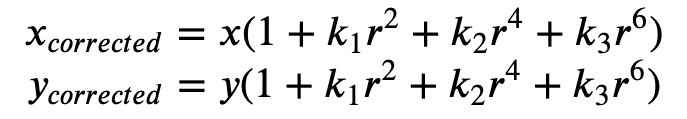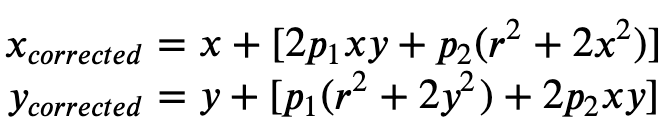• `k1``k2``k3``k4`— 径向畸变系数。鱼眼和针孔摄像机型号支持。

• `p1``p2`— 切向畸变系数。针孔摄像机型号支持。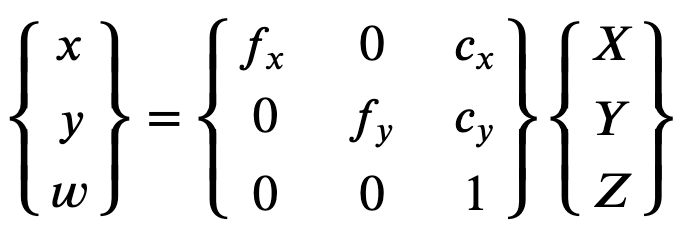• `fx` - x 方向的焦距。

• `fy` - y 方向的焦距。

• `cx` - 主点的 x 坐标。

• `cy` - 主点的 y 坐标。

``````def generate_view_matrix(intrinsic_matrix, extrinsic_matrix):
intrinsic_matrix = np.c_[intrinsic_matrix, np.zeros(3)]
view_matrix = np.matmul(intrinsic_matrix, extrinsic_matrix)
view_matrix = np.insert(view_matrix, 2, np.array((0, 0, 0, 1)), 0)
return view_matrix``````